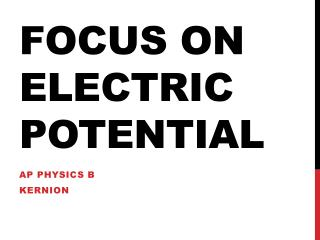DownloadDownload PresentationFocus on Electric Potential

# Focus on Electric Potential

Télécharger la présentation## Focus on Electric Potential

- - - - - - - - - - - - - - - - - - - - - - - - - - - E N D - - - - - - - - - - - - - - - - - - - - - - - - - - -
##### Presentation Transcript

1. Focus on Electric Potential AP Physics B Kernion

2. Main Points • Electrical Potential Energy (UE), scalar quantity depends on: • position in the E-field • amount of charge • magnitude of the E-field at that point • For uniform E-fields: • ΔU = -qEd for a positive charge moving ALONG the field • ΔU = qEdfor a negative charge moving ALONG the field • A new scalar quantity, Electric potential (V) is defined as the electric potential energy per unit charge • U/q = V (You MUST use the sign on q) • ΔU/q = ΔV (The change in electric potential...voltage) • unit = J/C = volt (v)

3. For movement along a uniform E-field • For a positive charge • ΔU/q = ΔV = -qEd/q = -Ed • For a negative charge • ΔU/q = ΔV = qEd/-q = -Ed • Movement ALONG the field ALWAYS lowers the electrical potential • N/C = V/m

4. Example • A proton is released from rest in a uniform E-field (E = 8 x 104 V/m) directed to the right. The proton moves a distance of 0.5 m along the field. Find the following quantities • ΔV, ΔU,ΔK, v, WE • ΔV = -Ed = (8x104)(0.5) = 4x104 volts • ΔU = qΔV = 1.6x10-19C (4x104v) = 6.4x10-15J • ΔK = -ΔU = -6.4x10-15 J = ½ mv2 = ½(1.67x10-27kg) • v = 2.8x106m/s • WE = -ΔU = ΔK = -6.4x10-15 J

5. Electric Potential and non-uniform E-fields + Electric potential caused by a point particle V = kq/r (keep sign on charge)

6. Example Problem Find the electric potential at point p p 4 m 3 m q1 q2 (0.5 μC) (-2.0 μC) VP = ∑kq/r = k[q1/r1 + q2/r2] VP = 9x109[(0.5x10-6/4) + (-2x10-6/5) VP = -2475 volts